• 文章目录决策树CART算法决策树CART算法学习目标决策树CART算法详解基尼指数和熵CART算法对连续值特征的处理CART算法对离散值特征的处理CART算法剪枝生成剪枝后的决策树选择最优子树CART算法剪枝流程输入输出流程决策...
文章目录决策树CART算法决策树CART算法学习目标决策树CART算法详解基尼指数和熵CART算法对连续值特征的处理CART算法对离散值特征的处理CART算法剪枝生成剪枝后的决策树选择最优子树CART算法剪枝流程输入输出流程决策树CART算法流程输入输出分类CART树算法流程回归CART树算法流程处理连续值预测结果决策树CART算法优缺点优点缺点小结
决策树CART算法决策树C4.5算法虽然对决策树ID3算法做了很大的改良，但是缺点也是很明显的，无法处理回归问题、使用较为复杂的熵来作为特征选择的标准、生成的决策树是一颗较为复杂的多叉树结构，CART算法针对这些问题又做了进一步的优化。
决策树CART算法学习目标

基尼指数和熵
CART算法对连续值和特征值的处理
CART算法剪枝
决策树CART算法的步骤
决策树CART算法的优缺点

决策树CART算法详解
CART的英文名全称是classification and regression tree，所以有时候也把CART称它为分类回归树，分类回归树由特征选择、树的生成以及剪枝组成，既可以用于分类也可以用于回归。
基尼指数和熵
# 基尼指数和熵示例图
import numpy as np
import matplotlib.pyplot as plt
from matplotlib.font_manager import FontProperties
%matplotlib inline
font = FontProperties(fname='/Library/Fonts/Heiti.ttc')

p = np.arange(0.001, 1, 0.001)
gini = 2*p*(1-p)
entropy = -(p*np.log2(p) + (1-p)*np.log2(1-p))/2
error = 1-np.max(np.vstack((p, 1-p)), 0)
plt.plot(p, entropy, 'r-', label='基尼指数')
plt.plot(p, gini, 'g-', label='熵之半$(1/2*H(p))$')
plt.plot(p, error, 'b-', label='分类误差率')
plt.xlabel('p', fontproperties=font)
plt.ylabel('损失', fontproperties=font)
plt.legend(prop=font)
plt.show()

[外链图片转存失败,源站可能有防盗链机制,建议将图片保存下来直接上传(img-blnb0GBe-1582805411133)(02-23%20%E5%86%B3%E7%AD%96%E6%A0%91CART%E7%AE%97%E6%B3%95_files/02-23%20%E5%86%B3%E7%AD%96%E6%A0%91CART%E7%AE%97%E6%B3%95_7_0.png)]
上图可以看出二分类问题中基尼指数和熵的曲线非常接近，因此基尼指数可以作为熵的一个近似替代。而CART算法就是使用了基尼指数来选择决策树的特征，同时为了进一步简化基尼指数的计算，CART算法每次对某个特征进行二分，因此CART算法构造的决策树是一颗二叉树模型。
CART算法对连续值特征的处理
CART算法类似于C4.5算法对连续值特征的处理，只是CART算法使用基尼指数取代了信息增益比对连续值做了处理。
假设现有一个特征$F$的特征值为连续值，从大到小排序为$f_1,f_2,\ldots,f_m$，CART算法对相邻样本间的特征值$f_i,f_{i+1}$取平均数，一共可以得到$m-1$个划分点，其中第$j$个划分点可以表示为
$S_j = {\frac {f_i + f_{i+1}} {2}}$
对于这$m-1$个划分点，分别计算以该点作为二元分类点的基尼指数，选择基尼指数最小的点作为该连续特征的二元离散分类点，把改点记作$f_t$，则特征值小于$f_t$的点记作$c_1$；特征值大于$f_t$的点记作$c_2$，这样就实现了连续特征值的离散化。
CART算法对离散值特征的处理
CART算法对离散值特征的处理采用的是不停的二分离散化特征的思想。
假设一个训练集$D$的某个特征$F$有$f_1,f_2,f_3$三种类别。如果我们使用的是ID3算法或者是C4.5算法，则会生成$3$个子节点，即三叉子节点，也因此导致决策树变成一颗多叉树。但是CART算法会基于这三个特征形成$f_1$和$f_2,f_3$、$f_2$和$f_1,f_3$、$f_3$和$f_1,f_2$这三种组合，并且在这三个组合中找到基尼指数最小的组合，然后生成二叉子节点。
假设$f_1$和$f_2,f_3$在这三者中基尼指数最小，则生成的二叉做子节点为$f_1$，二叉右子节点为$f_2,f_3$。由于右子节点并没有被完全分开，因此在之后会继续求出$f_2$和$f_3$的基尼指数，然后找到最小的基尼指数来划分特征$F$。
CART算法剪枝
回归CART树和分类CART树剪枝策略除了在特征选择的时候一个使用了均方误差，另一个使用了基尼指数，其他内容都一样。
无论是C4.5算法还是CART算法形成的决策树都很容易对训练集过拟合，因此可以使用剪枝的方式解决过拟合问题，这类似于线性回归中的正则化。
CART算法采用的事后剪枝法，即先生成决策树，然后产生所有可能的剪枝后的CART树，然后使用交叉验证来检验各种剪枝的效果，选择返回泛化能力最好的剪枝方法。即CART树的剪枝方法可分为两步：

使用原始的决策树$T_0$从它的底端开始不断剪枝，直到$T_0$的根节点生成一个子树序列$\{T_0,T_1,\ldots,T_n\}$
通过交叉验证法选择泛化能力最好的剪枝后的树作为最终的CART树，即最优子树

生成剪枝后的决策树
在剪枝过程中，子树$T$的损失函数为
$C_\alpha(T) = C(T) + \alpha|T|$
其中$T$是任意子树，$\alpha \quad \alpha\geq0$为正则化参数，它权衡训练数据的拟合程度与模型的复杂度；$C(T)$是训练数据的预测误差（分类树使用基尼指数度量，回归树使用均方差度量），$|T|$是子树$T$的叶子节点的数量。
当$\alpha=0$时没有正则化，即原始的决策树为最优子树；当$\alpha$逐渐增大时，则正则化强度越大，生成的最优子树相比较原生的子树就越小；当$\alpha=\infty$时，即正则化强度达到最大，此时由原始的决策树的根节点组成的单节点树为最优子树。因此对于固定的$\alpha$，从子树的损失函数中可以看出一定存在使损失函数$C_\alpha(T)$最小的唯一子树$T_a$，$T_a$在损失函数最小的意义下是最优的。
可以递归的方法对书进行剪枝。将$\alpha$从小增大，$0=\alpha_0<\alpha_1<\cdots\alpha_n<+\infty$，产生一系列的区间$[\alpha_i,\alpha_{i+1}),i=0,1,\ldots,n$；剪枝得到的子序列对应着区间$\alpha{\in}{[\alpha_i,\alpha_{i+1})}$的最优子树序列$\{T_0,T_1,\ldots,T_n\}$(注：每个区间内是有可能有多个子树的)，序列中的子树是嵌套的。
从原始的决策树$T_0$开始剪枝，对$T_0$的任意内部节点$t$，以$t$为单结点树的损失函数是
$C_\alpha(t) = C(t) + \alpha$
以$t$为根节点的子树$T_t$的损失函数是
$C_\alpha(T_t) = C(T_t) + \alpha|T_t|$
当$\alpha=0$以及$\alpha$充分小时（最优子树为原始的决策树），有不等式
$C_\alpha(T_t) < C_\alpha(t)$
当$\alpha$增大时，在某一$\alpha$有
$C_\alpha(T_t) = C_\alpha(t)$
当$\alpha$继续增大时（最优子树为根节点组成的单节点树），有
$C_\alpha(T_t) > C_\alpha(t)$
并且只要当$\alpha = {\frac {C(t)-C(T_t)} {|T_t|-1} }$(注：当$T_t$和$t$有相同的损失函数时该公式由$t$和$T_t$的损失函数联立得到)。由于$t$的节点少，因此$t$比$T_t$更可取，因此可以对子树$T_t$剪枝，也就是将它的子节点全部剪掉，变为一个叶子节点$t$。
选择最优子树
上面说到可以计算出每个子树是否剪枝的阈值$\alpha$，如果把所有的节点是否剪枝的值$\alpha$都计算出来，然后分别针对不同的$\alpha$所对应的剪枝后的最优子树做交叉验证，这样就可以选择一个最优的$\alpha$，通过这个$\alpha$则可以用对应的最优子树作为最终结果。
CART算法剪枝流程
输入
假设现在有一个原始的决策树$T_0$。
输出
最优子树$T_\alpha$。
流程

初始化$\alpha_{min}=\infty$，最优子树集合$s=\{T\}$
自下而上的对各内部结点$t$计算$C(T_t)$、$|T_t|$以及正则化阈值$\alpha = min\{\alpha_{min},g(t)={\frac{C(t)-C(T_t)}{|T_t|-1}}\}$(注：$g(t)$由$t$和$T_t$的损失函数联立得到，即表示剪枝后整体损失函数的减少程度)，并且更新$\alpha_{min}=\alpha$。其中$T_t$表示以$t$为根节点的子树，$C(T_t)$是训练数据的预测误差，$|T_t|$是$T_t$的叶节点个数
得到所有节点的$\alpha$值的集合$M$
从$M$中选择最大的值$\alpha_i$，自上而下的访问子树$t$的内部节点，如果${\frac{C(t)-C(T_t)}{|T_t|-1}}\leq\alpha_i$(注：$g(t)=C_\alpha(t)-C_\alpha(T_t)+\alpha$，如果$g(t)\leq\alpha$，则$C_\alpha(t)-C_\alpha(T_t)<0$，则$C_\alpha(t)，则以$t$为单节点的树的误差会更小)，进行剪枝并决定叶节点的值。如果是分类树，则是概率最高的类别；如果是回归树，则是所有样本输出的均值或所有样本的中位数。然后得到$\alpha_i$对应的最优子树$T_k$
最优子树集合$s=s\bigcup{T_i}$，$M=M-{a_i}$
如果$M$不为空，回到步骤4，否则已经得到了所有可能的最优子树集合$s$
采用交叉验证在$s$中选择最优子树$T_\alpha$决策树CART算法流程
输入
假设有训练数据集$D$，停止计算的条件：节点中的样本个数小于预定阈值，或样本集的基尼指数小于预定阈值，或没有更多特征。
输出
CART树
分类CART树算法流程
CART算法从根节点开始，用训练集递归的建立CART树。

设节点的训练集为$D$，计算现有的所有特征对该训练集的基尼指数。此时对每一个特征$F$，对其可能取的每个值$f$，根据样本点对$F=f$的测试为“是”或“否”将$D$分割成$D_1$和$D_2$两个子集，利用基尼指数公式计算$F=f$时的基尼指数。
在所有可能的特征$T$以及它们所有可能的切分点$t$中，选择基尼指数最小的特征与其对应的切分点作为最优切分点，依据该最优切分点切割，生成两个子节点，左子节点为$D_1$，右子节点为$D_2$。
对两个子节点递归调用步骤$1-2$，直至满足停止条件生成一颗完整的二叉CART决策树。
对生成的分类CART决策树做预测的时候，如果测试集里的某个样本$A$落到了某个叶子节点，该叶子节点里存在多个类别的训练样本，则概率最大的训练样本则是样本$A$的类别。

回归CART树算法流程
回归CART树和分类CART树的建立算法和过程大部分是相同的，所以本文只讨论两者生成决策树的区别，两者的区别有以下两点

回归CART树和分类CART树最大的区别在于样本输出，如果输出的是离散值，则它是一颗分类树；如果输出的是连续值，则它是一颗回归树。
两者的区别在于对连续值处理的方式不同以及决策树建立后做预测的方式不同。

处理连续值
分类CART树用基尼指数最小化准则，而回归CART树用均方误差最小化准则作为特征和划分点选择的方法。
对任意的划分特征$F$，对应的任意划分点$s$把训练集$D$划分成两个子集$D_1$和$D_2$，求出使得两个子集$D_1$和$D_2$以及两个子集之和的均方差最小的对应划分点$s$特征$F$和划分点$s$，即
$\underbrace{min}_{F,s} [ \underbrace{min}_{c_1} \sum_{{x_i}\in{D_1(F,s)}} (y_i-c_1)^2 + \underbrace{min}_{c_2} \sum_{{x_i}\in{D_2(F,s)}} (y_i-c_2)^2 ]$
其中已经假设样本按照某个特征$F$和划分点$s$划分成功，则输入一个$x$会有一个输出值$c_m$。$c_1$则是$D_1$数据集中所有的$x$的样本输出均值，$c_2$是$D_2$数据集中所有的$x$的样本输出均值。
使用该方法生成的回归CART树通常称作最小二乘回归树（least squares regression tree）。
预测结果
分类CART树选择概率最大的类别作为样本$A$的类别的方式不同的是：回归CART树由于输出的不是类别，而是一个连续值，因此它通常采用的是使用最终叶子的均值或者中位数来预测输出结果。
决策树CART算法优缺点
优点

既可以做分类又可以做回归

缺点

你要说缺点其实还真的有，CART算法做特征分类决策的时候是由某一个特征决定的，而真实情况应该是由一组特征决定的，这样决策得到的决策树更加准确，这个决策树叫做多变量决策树（mutil-variate decision tree），这个算法的代表是OC1，这里不多赘述。

小结
CART树是决策树的一次创新，摒弃了信息熵使用了基尼指数，基于C4.5算法可以处理回归问题，可以使用剪枝防止过拟合，解释型强。
CART树可以说是完美的，但是它最大的一个问题就是CART算法会把所有的特征全部用于构造决策树中，这对于生成决策树来讲是一个非常大的问题，在集成学习中使用随机森林将能一点程度的减轻该问题。
由于随机森林属于集成学习，所以下一篇很遗憾的告诉你不是讲随机森林，而将带你走入概率的天堂，即朴素贝叶斯法。展开全文• 这是我从网上找到的一份决策树Cart算法代码，其中在确定分枝时采用的是熵不纯度确定的方法,代码可以运行.声明这份代码不是我原创的，是从某个网页上下载下来的，不过原作者的代码中许多变量没有作详细注释，我在阅读...
• 决策树CART算法原理理解一、CART回归决策树算法原理（一）、回归树的生成最优特征及最优切分点的选择（二）、最小二乘回归树生成算法二、CART分类树算法原理（一）、分类树的生成最优特征及最优切分点的选择（二）、...
决策树CART算法原理理解一、CART回归决策树算法原理（一）、回归树的生成最优特征及最优切分点的选择（二）、最小二乘回归树生成算法二、CART分类树算法原理（一）、分类树的生成最优特征及最优切分点的选择（二）、分类树生成算法三、CART对于特征为连续值以及离散值的处理参考
首先应该清楚回归树与分类树的本质区别在于模型的输出值不同，如果输出值为连续值则为回归树，如果为离散值则为分类树。
一、CART回归决策树算法原理
（一）、回归树的生成
假设现在已有训练数据集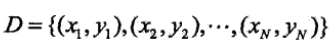并且假设已经将输入空间划分为M个单元R1,R2,……RM,此时如果给定输入空间的一个划分，那么回归树在这个划分上的预测误差即为（用平方误差表示如下）：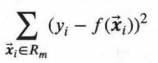那么（此处假设为基于平方误差最小的准则）此回归树模型在每个划分单元上的最优输出值即为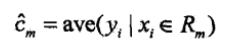上面公式的含义就是Rm单元内包括的样本对应的数据集中y值的平均值
最优特征及最优切分点的选择
那么现在的问题就在于依据什么原则去找到最佳的划分，现在假设样本共有k个特征（k维），假设现在选择第j维和它此时的取值为s，作为划分数据集的特征以及特征值（切分点），将数据集划分为两部分R1，R2: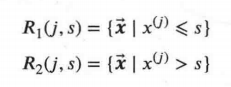我们选择最优特征以及最优切分点的准则就是：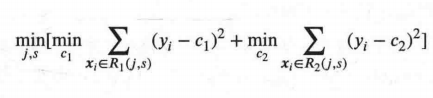怎么理解上面的式子呢，就是对于任意划分特征，对应的任意划分点s两边划分成的数据集R1和R2，求出使R1和R2内各自含有的样本的输出值的平方误差最小，同时两者平方误差之和最小所对应的特征j和特征值划分点s。
（二）、最小二乘回归树生成算法
这里直接贴上李航老师的描述：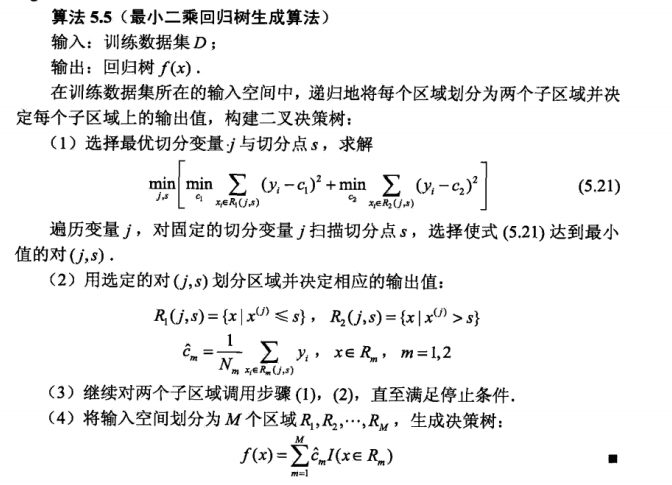这里补充一下一般停止条件有三种：
1.节点中的样本数量小于指定值
2.节点中样本的平方误差小于指定值
3.已经没有更多的特征
二、CART分类树算法原理
（一）、分类树的生成
首先需要知道的是基尼指数这个概念，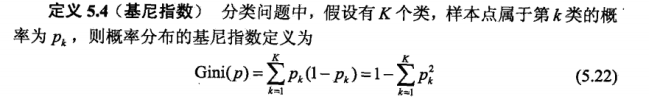基尼指数是用来表示不确定性的一个概念。
对于给定的样本数据集D,它的基尼指数定义为：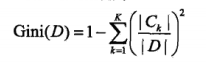此时对于样本数据集D,如果根据特征A是否取其某个值a,把D分成D1和D2两部分，则在特征A的条件下，D的基尼系数表达式为：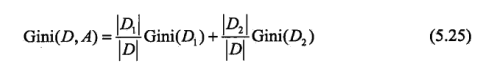Gini（D)表示数据集D的不确定性，Gini（D,A）表示在A=a分割后D的不确定性，基尼指数越大表明不确定性越大。
最优特征及最优切分点的选择
如何选择最优特征以及切分点呢？依据的原则就是选择的特征A以及它对应的取值a使得Gini（D，A=a)最小。
怎么理解这句话，举个小例子，现在D共有职业A,年龄B,性别C三个特征，其中A可取值为“老师”，“学生”，“其他”，B假设样本中取值就只有“20”，“30”，“40”，C可能取值为“男”，“女”
现在分别计算Gini(D,A="老师“），Gini(D,A="学生“），Gini(D,A="其他“），Gini(D,B="20“）,Gini(D,B="30“）,Gini(D,B="40“）,Gini(D,C="男“），Gini(D,C="女“），然后选出最小的，假设为Gini(D,A="学生“）最小那么这一轮的最优特征就为职业，最优划分点就为是否为学生。
（二）、分类树生成算法
这里直接贴上李航老师的描述：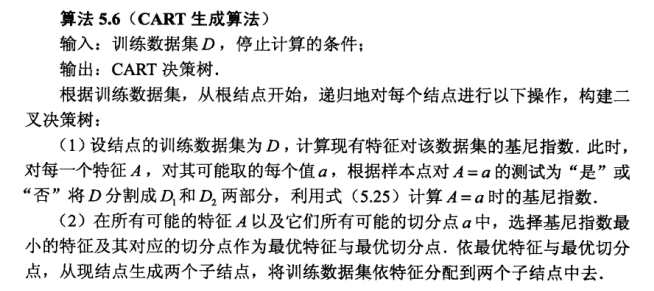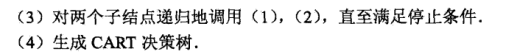这里补充一下一般停止条件有三种：
1.节点中的样本数量小于指定值
2.节点中样本的平方误差小于指定值
3.已经没有更多的特征
三、CART对于特征为连续值以及离散值的处理
首先，我们应该知道CART产生的是完全二叉树。
对于CART连续值的处理问题，其思想使将连续的特征离散化。
”具体的思路如下，比如m个样本的连续特征A有m个，从小到大排列为a1,a2,…,am,则CART算法取相邻两样本值的平均数，一共取得m-1个划分点，其中第i个划分点Ti表示为：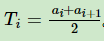对于这m-1个点，分别计算以该点作为二元分类点时的基尼系数。选择基尼系数最小的点作为该连续特征的二元离散分类点。比如取到的基尼系数最小的点为at,则小于at的值为类别1，大于at的值为类别2，这样我们就做到了连续特征的离散化。要注意的是，与ID3或者C4.5处理离散属性不同的是，如果当前节点为连续属性，则该属性后面还可以参与子节点的产生选择过程。“
对于特征取值为离散值的处理问题，CART采用的思路是不停的二分离散特征。
回忆下ID3或者C4.5，如果某个特征A被选取建立决策树节点，如果它有A1,A2,A3三种类别，我们会在决策树上一下建立一个三叉的节点。这样导致决策树是多叉树。但是CART分类树使用的方法不同，他采用的是不停的二分，还是这个例子，CART分类树会考虑把A分成{A1}和{A2,A3}, {A2}和{A1,A3}, {A3}和{A1,A2}三种情况，找到基尼系数最小的组合，比如{A2}和{A1,A3},然后建立二叉树节点，一个节点是A2对应的样本，另一个节点是{A1,A3}对应的节点。同时，由于这次没有把特征A的取值完全分开，后面我们还有机会在子节点继续选择到特征A来划分A1和A3。这和ID3或者C4.5不同，在ID3或者C4.5的一棵子树中，离散特征只会参与一次节点的建立。
对于CART分类树离散值的处理问题，采用的思路是不停的二分离散特征。
回忆下ID3或者C4.5，如果某个特征A被选取建立决策树节点，如果它有A1,A2,A3三种类别，我们会在决策树上一下建立一个三叉的节点。这样导致决策树是多叉树。但是CART分类树使用的方法不同，他采用的是不停的二分，还是这个例子，CART分类树会考虑把A分成{A1}和{A2,A3}, {A2}和{A1,A3}, {A3}和{A1,A2}三种情况，找到基尼系数最小的组合，比如{A2}和{A1,A3},然后建立二叉树节点，一个节点是A2对应的样本，另一个节点是{A1,A3}对应的节点。同时，由于这次没有把特征A的取值完全分开，后面我们还有机会在子节点继续选择到特征A来划分A1和A3。这和ID3或者C4.5不同，在ID3或者C4.5的一棵子树中，离散特征只会参与一次节点的建立。"这段话是刘建平老师博客里的讲解，其实对于回归树同样适用，不同的是依据使平方误差和最小的准则去选择最优特征和最优切分点
参考
李航《统计学习方法》
刘建平老师博客


展开全文• 机器学习：决策树cart算法在分类与回归的应用（下）-附件资源
•  Classification And Regression Tree，即分类回归树算法，简称CART算法，它是决策树的一种实现，通常决策树主要有三种实现，分别是ID3算法，CART算法和C4.5算法。  CART算法是一种二分递归分割技术，把当前样本...
1. CART算法的认识

Classification And Regression Tree，即分类回归树算法，简称CART算法，它是决策树的一种实现，通常决策树主要有三种实现，分别是ID3算法，CART算法和C4.5算法。
CART算法是一种二分递归分割技术，把当前样本划分为两个子样本，使得生成的每个非叶子结点都有两个分支，
因此CART算法生成的决策树是结构简洁的二叉树。由于CART算法构成的是一个二叉树，它在每一步的决策时只能是“是”或者“否”，即使一个feature有多个取值，也是把数据分为两部分。在CART算法中主要分为两个步骤
（1）将样本递归划分进行建树过程
（2）用验证数据进行剪枝
2. CART算法的原理

上面说到了CART算法分为两个过程，其中第一个过程进行递归建立二叉树，那么它是如何进行划分的 ？
设代表单个样本的个属性，表示所属类别。CART算法通过递归的方式将维的空间划分为不重叠的矩形。划分步骤大致如下
（1）选一个自变量，再选取的一个值，把维空间划分为两部分，一部分的所有点都满足，另一部分的所有点都满足，对非连续变量来说属性值的取值只有两个，即等于该值或不等于该值。
（2）递归处理，将上面得到的两部分按步骤（1）重新选取一个属性继续划分，直到把整个维空间都划分完。
在划分时候有一个问题，它是按照什么标准来划分的 ？ 对于一个变量属性来说，它的划分点是一对连续变量属
性值的中点。假设个样本的集合一个属性有个连续的值，那么则会有个分裂点，每个分裂点为相邻
两个连续值的均值。每个属性的划分按照能减少的杂质的量来进行排序，而杂质的减少量定义为划分前的杂质减
去划分后的每个节点的杂质量划分所占比率之和。而杂质度量方法常用Gini指标，假设一个样本共有类，那么一个节点的Gini不纯度可定义为其中表示属于类的概率，当Gini(A)=0时，所有样本属于同类，所有类在节点中以等概率出现时，Gini(A)
最大化，此时。

有了上述理论基础，实际的递归划分过程是这样的：如果当前节点的所有样本都不属于同一类或者只剩下一个样
本，那么此节点为非叶子节点，所以会尝试样本的每个属性以及每个属性对应的分裂点，尝试找到杂质变量最大
的一个划分，该属性划分的子树即为最优分支。

下面举个简单的例子，如下图在上述图中，属性有3个，分别是有房情况，婚姻状况和年收入，其中有房情况和婚姻状况是离散的取值，而年
收入是连续的取值。拖欠贷款者属于分类的结果。

假设现在来看有房情况这个属性，那么按照它划分后的Gini指数计算如下而对于婚姻状况属性来说，它的取值有3种，按照每种属性值分裂后Gini指标计算如下最后还有一个取值连续的属性，年收入，它的取值是连续的，那么连续的取值采用分裂点进行分裂。如下根据这样的分裂规则CART算法就能完成建树过程。
建树完成后就进行第二步了，即根据验证数据进行剪枝。在CART树的建树过程中，可能存在Overfitting，许多分支中反映的是数据中的异常，这样的决策树对分类的准确性不高，那么需要检测并减去这些不可靠的分支。决策
树常用的剪枝有事前剪枝和事后剪枝，CART算法采用事后剪枝，具体方法为代价复杂性剪枝法。

原文参考：http://blog.csdn.net/acdreamers/article/details/44664481
剪枝参考：http://www.cnblogs.com/zhangchaoyang/articles/2709922.html


展开全文• 这是一个使用matlab来实现决策树cart算法
• 决策树CART算法——基尼系数 决策树的CART算法使用基尼系数来选择划分属性。一个数据集的纯度可以用基尼系数来度量 Gini(D)=∑k=1∣y∣∑k′≠kpkpk′=1−∑k=1∣y∣pk2\begin{aligned}Gini(D) = \sum_{k=1}^{|y|}\...
决策树CART算法——基尼系数
决策树的CART算法使用基尼系数来选择划分属性。一个数据集的纯度可以用基尼系数来度量
\begin{aligned}Gini(D) = \sum_{k=1}^{|y|}\sum_{k'\ne k}p_kp_{k'} = 1-\sum_{k=1}^{|y|}p_k^2\end{aligned}
直观来说，数据集的基尼系数反映了从数据集D中随机抽取两个样本，其类别不一样的概率。因此，基尼系数越小，数据集的纯度越高。
那么属性a的基尼系数为
\begin{aligned}Gini\_index(D,a) = \sum_{v=1}^{V}\frac{|D^v|}{D}Gini(D^v)\end{aligned}
与数据集D中a属性的熵值计算类似，参考我的博文1和我的博文2
于是，我们在候选属性集合A中，选择那个使得划分后的基尼指数值最小的属性作为最优划分属性
$a_* = argmin_{a\in A}Gini\_index(D,a)$


展开全文• 大家好，今天用一个简单的例子来给大家介绍一下决策树中的CART算法。 CART分类树 CART分类树适用于预测结果为离散型数据的情况下，主要是计算每一组特征的Gini系数增益来确定决策树划分的优先规则，主要是采用一种...
• CART假设决策树是二叉树，内部结点特征的取值为“是”和“否”，左分支是取值为“是”的分支，右分支是取值为“否”的分支。这样的决策树等价于递归地二分每个特征，将输入空间即特征空间划分为有限个单元，并在这些...
•机器学习 python
• 前面，小编和大家一起学习了关于决策树C5.0算法进行决策树分析，今天，继续学习使用CART算法进行决策树分析。 首先，我们了解一下CART算法与C5.0算法的区别： C5.0算法只能处理分类型目标变量，CART算法既能处理...
• ID3和C4.5在上一篇，点击 转载：https://www.cnblogs.com/pinard/p/6053344.html
• 决策树算法包括ID3，C4.5，CART。这里的CART：classification and regression tree.CART的本质是对特征空间进行二元分割，即CART生成的树是一颗二叉树，并能对标称属性与数值型属性进行分割。 1. CART综述  树模型 ...机器学习
• 1.CART生成 CART假设决策树是二叉树，内部结点特征的取值为“是”和“否”，左分支是取值为“是”的分支，右...CART算法由以下两步组成： 决策树生成：基于训练数据集生成决策树，生成的决策树要尽量大； 决...
• 和之前介绍的ID3和C4.5一样，CART算法同样是决策树模型的一种经典的实现。决策树这个模型一共有三种实现方式，前面我们已经介绍了ID3和C4.5两种，今天刚好补齐这最后一种。 算法特点 CART称为分类回归树，从名字上...机器学习 Python 分类模型
• 决策树分为分类树和回归树两种，分类树对离散变量做决策树，回归树对连续变量做决策树，分类树是从ID3算法开始，改进成C4.5，随后又出现了cart算法cart算法可以生成分类树，也可以生成回归树，每个决策树之所以...机器学习
• %构建CART算法分类 tree=fitctree(X_train,Y_train); view(tree,'Mode','graph');%生成图 rules_num=(tree.IsBranchNode==0); rules_num=sum(rules_num);%求取规则数量 Cart_result=predict(tree,X_test);%...Matlab实现
• 先生成数据，再调用cart算法，生成决策树。 #生成模板数据 dataSet,labels=createSet(url) #复制标签 测试决策树时，原有标签对象不可用 labels_tmp=labels[:] #用决策树 desicionTree = createTree(dataSet,...
• CART决策树分类算法VC++ CART决策树分类算法VC++ CART决策树分类算法VC++ CART决策树分类算法VC++
•机器学习 数学
• CART算法的数学详细计算步骤，以及CART算法生成决策树的过程，可以详见：【机器学习】【决策树CART算法，用样本集一步一步详解如何求：基尼指数，最优特征，最优切分点本章仅提供CART算法的python实现代码，以...
• 我们可以把决策树分为 ID3 算法、C4.5 算法CART 算法。今天我来带你学习 CART 算法CART 算法，英文全称叫做 Classification And Regression Tree，中文叫做分类回归树。ID3 和 C4.5 算法可以生成二叉树或多叉树...
• 原文出自：ACdreamers-决策树CART算法 在之前介绍过决策树的ID3算法实现，今天主要来介绍决策树的另一种实现，即CART算法。   Contents    1. CART算法的认识  2. CART算法的原理 ...
• 文章目录CART算法1....与ID3和C4.5只有决策树的生成不同的是，CART算法由以下两步组成： （1）决策树生成：基于训练数据集生成一棵尽量大的决策树。 （2）决策树剪枝：用验证数据集对已生成的树......